# Area of a triangleA triangle is a geometric figure that has three points that do not lie on one straight line and three line segments that connect these points in pairs. The points of a triangle are called its vertices, and the segments are called its sides. Our online calculator will help you calculate the area of ​​a triangle in just a few seconds. To do this, you need to enter certain data, namely the length of its base, which is designated by the Latin letter "a" and the height of the triangle, which is designated by the Latin letter "h". The area of ​​the triangle is calculated by the formula:which means that the area of ​​a triangle is equal to the product of the length of its base and the height divided by two.

Remembering geometry: formulas for arbitrary, rectangular, isosceles and equilateral figures.

## How to find the area of ​​any triangle

There are different ways to calculate the area of ​​a triangle. Choose a formula depending on the quantities you know.

### Knowing the side and the height

1. Multiply the side of the triangle by the height drawn to that side.
2. Divide the result by two.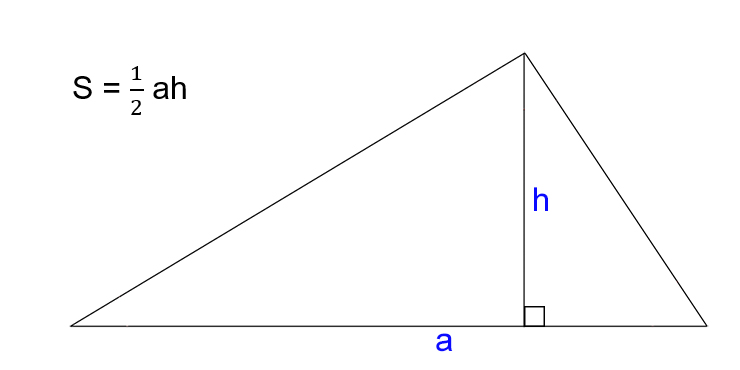• S is the required area of ​​the triangle.
• a - side of the triangle.
• h is the height of the triangle. This is a perpendicular dropped to the side or its extension from the opposite vertex.

### Knowing the two sides and the angle between them

1. Count the product of the two known sides of the triangle.
2. Find the sine of the angle between the selected sides.
4. Divide the result by two.• S is the required area of ​​the triangle.
• a and b are the sides of the triangle.
• α is the angle between sides a and b.

### Knowing the three sides (Heron's formula)

1. Calculate the differences between the half-perimeter of the triangle and each of its sides.
2. Find the product of the resulting numbers.
3. Multiply the result by a semi-perimeter.
4. Find the root of the resulting number.• S is the required area of ​​the triangle.
• a, b, c - sides of the triangle.
• p - half-perimeter (equal to half of the sum of all sides of the triangle).

### Knowing the three sides and the radius of the circumscribed circle

1. Find the product of all sides of the triangle.
2. Divide the result by the four radii of the circle around the rectangle.• S is the required area of ​​the triangle.
• R is the radius of the circumscribed circle.
• a, b, c - sides of the triangle.

### Knowing the radius of the inscribed circle and the semi-perimeter

Multiply the radius of the circle inscribed in the triangle by the semi-perimeter.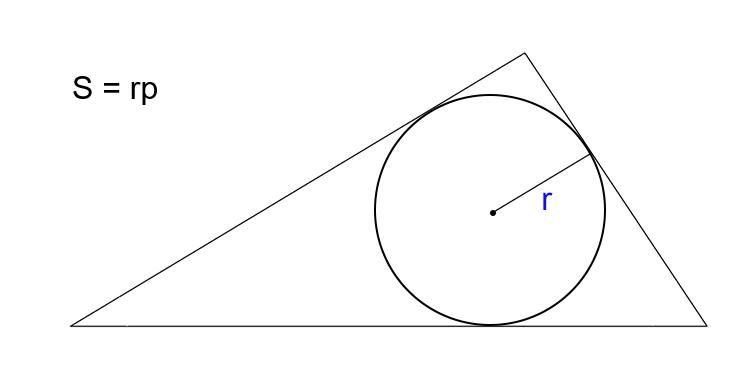• S is the required area of ​​the triangle.
• r is the radius of the inscribed circle.
• p - half-perimeter of a triangle (equal to half of the sum of all sides).

## How to find the area of ​​a right triangle

1. Count the product of the legs of the triangle.
2. Divide the result by two.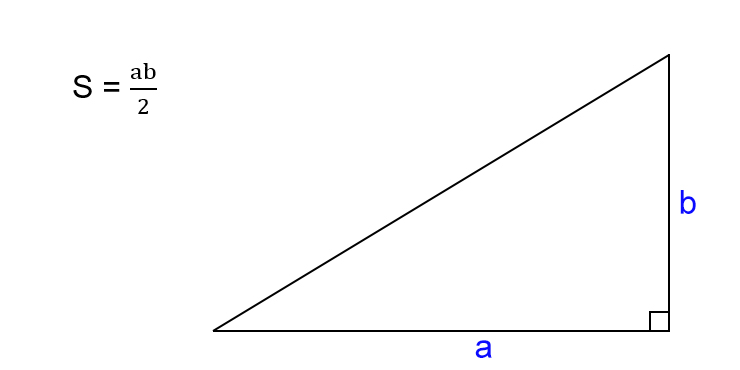• S is the required area of ​​the triangle.
• a, b - the legs of the triangle, that is, the sides that intersect at right angles.

## How to find the area of ​​an isosceles triangle

1. Multiply the base by the height of the triangle.
2. Divide the result by two.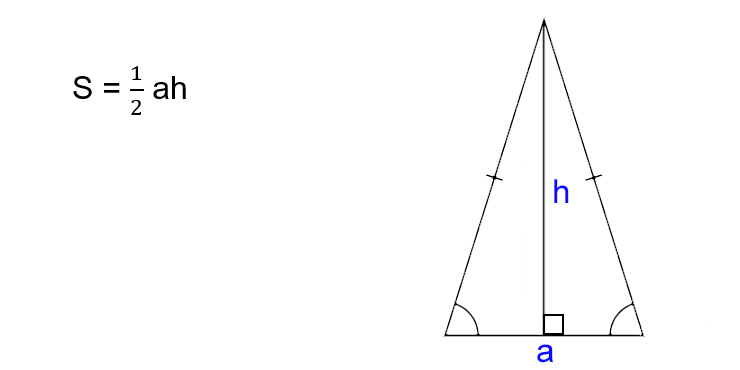• S is the required area of ​​the triangle.
• a is the base of the triangle. This is the side that is not equal to the other two. Recall that in an isosceles triangle, two of the three sides have the same length.
• h is the height of the triangle. It is a perpendicular dropped to the base from the opposite vertex.

## How to find the area of ​​an equilateral triangle

1. Multiply the square of the side of the triangle by the root of three.
2. Divide the result by four.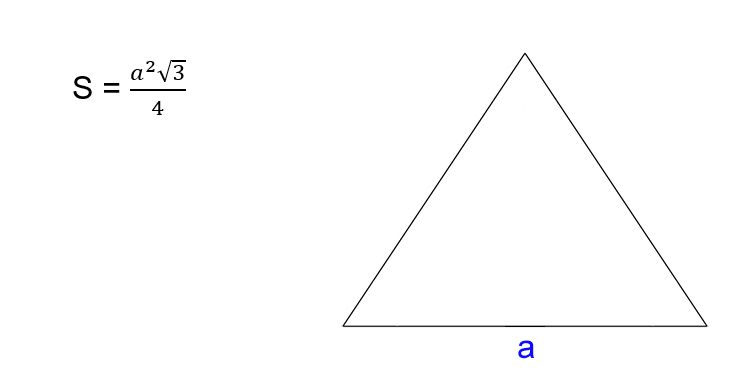• S is the required area of ​​the triangle.
• a - side of the triangle. Recall that in an equilateral triangle, all sides have the same length.

The online triangle area calculator will help you find the area of ​​a triangle in several ways, depending on the known data. Our calculator will not only calculate the area of ​​the triangle, but will also show you a detailed solution, which will be shown below the calculator. Therefore, this calculator is convenient to use not only for quick calculations, but also for checking your calculations. With this calculator, you can find the area of ​​a triangle using the following formulas: through the base and height, through two sides and an angle, along three sides (Heron's formula), through the radius of the inscribed circle, through the radius of the circumscribed circle.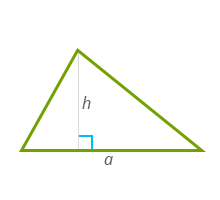Choose a method for calculating the area:

Calculate

A triangle is a geometric shape that is formed by three line segments. These segments are called the sides of the triangles, and the points of connection of the segments are called the vertices of the triangle. Depending on the aspect ratio, triangles are of several types: an isosceles triangle (two sided triangles are equal to each other, these sides are called lateral sides, and the third side is called the base of the triangle), an equilateral triangle (all three sides of a triangle are equal), a right-angled triangle (one angle a straight triangle).

## How do you find the area of ​​a triangle?

Finding the area of ​​a triangle is very simple, just use our calculator or calculate it yourself using the formula for the area of ​​a triangle. Depending on what data are known, several methods are used to calculate the area of ​​a triangle:

### 1) through the base and height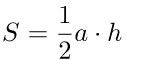a - the base of the triangle,

### h is the height of the triangle.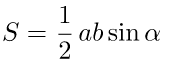2) through two sides and a corner

### a, b - sides of the triangle,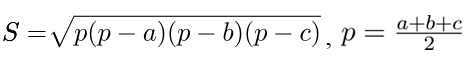α is the angle between the sides.

### 3) On three sides. Heron's formula.a, b, c - sides of the triangle,

### p is the semi-perimeter of the triangle.4) Through the radius of the inscribed circle.

a, b, c - sides of the triangle,

p is the semiperimeter of the triangle,

## 5) Through the radius of the circumscribed circle.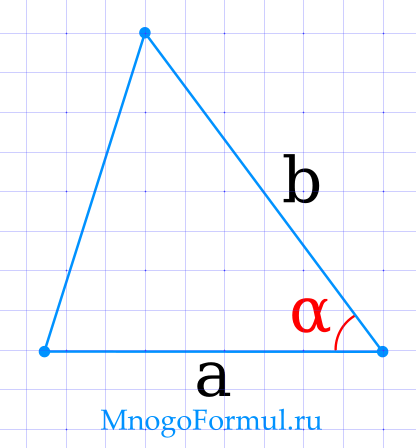a, b, c - sides of the triangle,

R is the radius of the circumscribed circle.

a, b, c - sides of the triangle, You can always check the correctness of calculating the area of ​​a triangle using our calculator. a, bThe task of finding the area of ​​a triangle is quite common not only in science, but also in everyday life. For you, we have developed 21 calculators for finding the area of ​​any triangle - isosceles, equilateral, rectangular or ordinary. αArea of ​​a triangle

## Area of ​​a triangle across two sides and the angle between them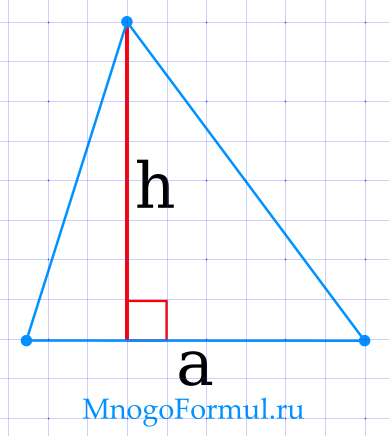{S = \ dfrac {1} {2} \ cdot a \ cdot b \ cdot sin (\ alpha)}

Formula for finding the area of ​​a triangle through 2 sides and an angle:

{S = \ dfrac {1} {2} \ cdot a \ cdot b \ cdot sin (\ alpha)} You can always check the correctness of calculating the area of ​​a triangle using our calculator. awhere h- sides of a triangle,

## - the angle between them.Area of ​​a triangle through base and height

{S = \ dfrac {1} {2} \ cdot a \ cdot h}

Area of ​​a triangle through base and height You can always check the correctness of calculating the area of ​​a triangle using our calculator. a, b, cThe task of finding the area of ​​a triangle is quite common not only in science, but also in everyday life. For you, we have developed 21 calculators for finding the area of ​​any triangle - isosceles, equilateral, rectangular or ordinary. RFormula for finding the area of ​​a triangle in terms of base and height:

## - the base of the triangle,Is the height of the triangle.

Area of ​​a triangle through the radius of the circumscribed circle and 3 sides

Is the height of the triangle. You can always check the correctness of calculating the area of ​​a triangle using our calculator. a, b, cThe task of finding the area of ​​a triangle is quite common not only in science, but also in everyday life. For you, we have developed 21 calculators for finding the area of ​​any triangle - isosceles, equilateral, rectangular or ordinary. r{S = \ dfrac {a \ cdot b \ cdot c} {4 \ cdot R}}

Formula for finding the area of ​​a triangle through the circumcircle and sides: Is the radius of the circumscribed circle. Area of ​​a triangle through the radius of the inscribed circle and 3 sides {S = r \ cdot \ dfrac {a + b + c} {2}} You can always check the correctness of calculating the area of ​​a triangle using our calculator. pFormula for finding the area of ​​a triangle in terms of the inscribed circle and sides:

## Is the radius of the inscribed circle.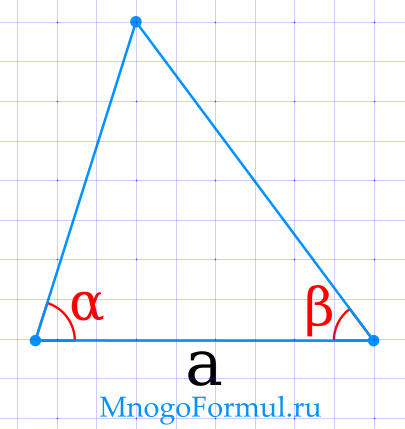The formula can be rewritten differently if we take into account that {\ dfrac {a + b + c} {2}}

- semi-perimeter of a triangle. In this case, the formula will look like this:

The formula can be rewritten differently if we take into account that You can always check the correctness of calculating the area of ​​a triangle using our calculator. aS = {r \ cdot p} α и β- semi-perimeter of a triangle. γArea of ​​a triangle through a side and two adjacent corners

{S = \ dfrac {a ^ 2} {2} \ cdot \ dfrac {sin (\ alpha) \ cdot sin (\ beta)} {sin (\ gamma)}}

## {\ gamma = 180 - (\ alpha + \ beta)}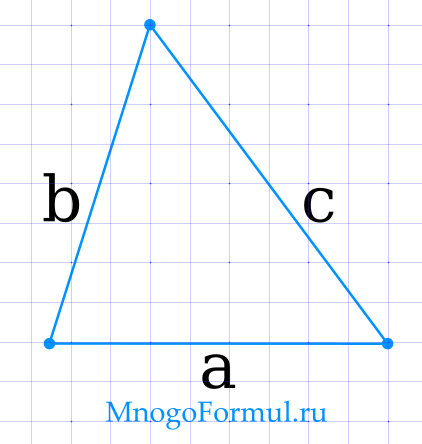Formula for finding the area of ​​a triangle in terms of the side and 2 adjacent angles: - side of the triangle,

Formula for finding the area of ​​a triangle in terms of the side and 2 adjacent angles: You can always check the correctness of calculating the area of ​​a triangle using our calculator. a, b, cThe task of finding the area of ​​a triangle is quite common not only in science, but also in everyday life. For you, we have developed 21 calculators for finding the area of ​​any triangle - isosceles, equilateral, rectangular or ordinary. p- the opposite angle, which can be found by the formula: {\ gamma = 180 - (\ alpha + \ beta)}

## {S = \ sqrt {p \ cdot (p-a) \ cdot (p-b) \ cdot (p-c)}}{p = \ dfrac {a + b + c} {2}}

Formula for finding the area of ​​a triangle using Heron's formula (if 3 sides are known):

{p = \ dfrac {a + b + c} {2}} You can always check the correctness of calculating the area of ​​a triangle using our calculator. a, bIs the semi-perimeter of a triangle, which can be found by the formula

## p = {\ dfrac {a + b + c} {2}}Area of ​​a right triangle

Area of ​​a right triangle across 2 sides

Area of ​​a right triangle You can always check the correctness of calculating the area of ​​a triangle using our calculator. c{S = \ dfrac {1} {2} \ cdot a \ cdot b} αFormula for finding the area of ​​a right-angled triangle on two sides:

## - the sides of the triangle.Area of ​​a right triangle through hypotenuse and acute angle

{S = \ dfrac {1} {4} \ cdot c ^ 2 \ cdot sin (2 \ alpha)}

Area of ​​a right triangle through hypotenuse and acute angle You can always check the correctness of calculating the area of ​​a triangle using our calculator. aFormula for finding the area of ​​a right-angled triangle by hypotenuse and acute angle: α- the hypotenuse of the triangle,

## - any of the adjacent sharp corners.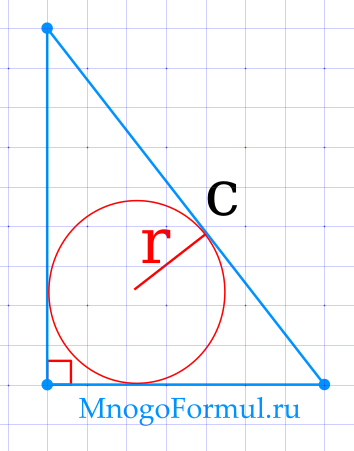Area of ​​a right triangle through the leg and included angle

{S = \ dfrac {1} {2} \ cdot a ^ 2 \ cdot tg (\ alpha)}

Formula for finding the area of ​​a right-angled triangle by leg and adjacent angle: You can always check the correctness of calculating the area of ​​a triangle using our calculator. c{S = \ dfrac {1} {2} \ cdot a \ cdot b} r{S = \ dfrac {a \ cdot b \ cdot c} {4 \ cdot R}}

## - leg of the triangle,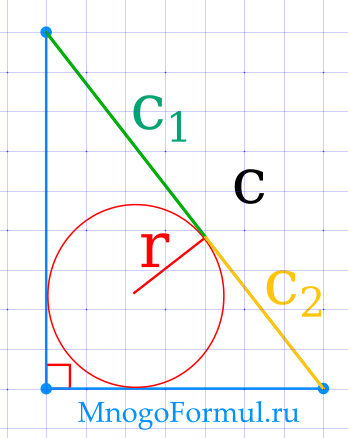- the included angle.

Area of ​​a right triangle through the radius of the inscribed circle and the hypotenuse

- the included angle. You can always check the correctness of calculating the area of ​​a triangle using our calculator. c1 и c2{S = r \ cdot (r + c)}

## Formula for finding the area of ​​a right-angled triangle by the radius of the inscribed circle and the hypotenuse: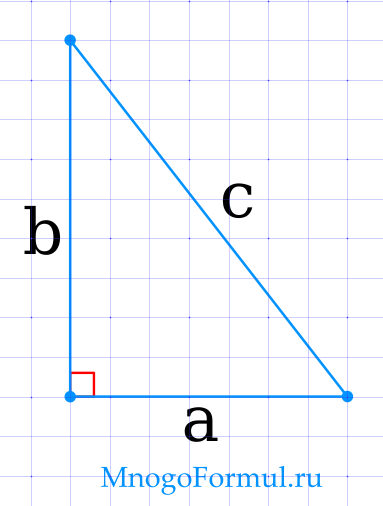{S = r \ cdot (r + c)} - side of the triangle,

Area of ​​a right triangle through the inscribed circle

{S = r \ cdot (r + c)} You can always check the correctness of calculating the area of ​​a triangle using our calculator. a, b{S = c_ {1} \ cdot c_ {2}} pFormula for finding the area of ​​a right-angled triangle along the inscribed circle: {\ gamma = 180 - (\ alpha + \ beta)}

## Area of ​​a right-angled triangle according to Heron's formula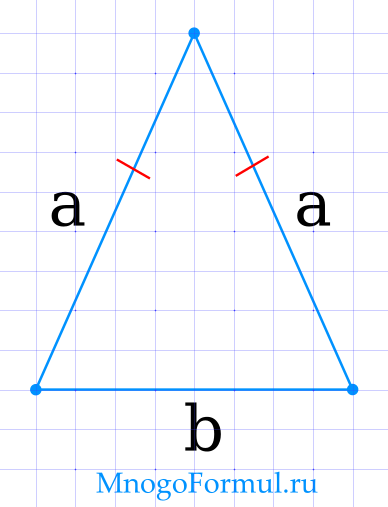{S = (p-a) \ cdot (p-b)}

Heron's formula for a right-angled triangle looks like this:

{S = (p-a) \ cdot (p-b)} You can always check the correctness of calculating the area of ​​a triangle using our calculator. a- the legs of the triangle, b- semi-perimeter of a right-angled triangle, which is calculated by the formula

## Area of ​​an isosceles triangleArea of ​​an isosceles triangle through the base and side

{S = \ dfrac {b} {4} \ sqrt {4 \ cdot a ^ 2-b ^ 2}}

Area of ​​an isosceles triangle through the base and side You can always check the correctness of calculating the area of ​​a triangle using our calculator. a- the legs of the triangle, bwhere αFormula for the area of ​​an isosceles triangle in terms of base and side:

## - the side of the triangle,- the base of the triangle

Area of ​​an isosceles triangle through base and angle

- the base of the triangle You can always check the correctness of calculating the area of ​​a triangle using our calculator. bwhere h{S = \ dfrac {1} {2} \ cdot a \ cdot b \ cdot sin (\ alpha)}

## Formula for the area of ​​an isosceles triangle in terms of the base and angle: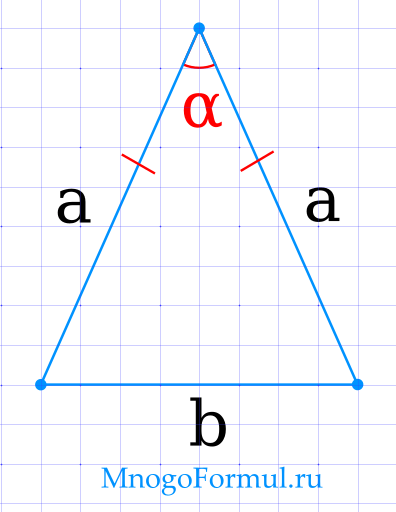- the angle between the base and the side.

Area of ​​an isosceles triangle through base and height

- the angle between the base and the side. You can always check the correctness of calculating the area of ​​a triangle using our calculator. a- the legs of the triangle, α{S = \ dfrac {1} {2} \ cdot b \ cdot h}

## Formula for the area of ​​an isosceles triangle in terms of the base and height: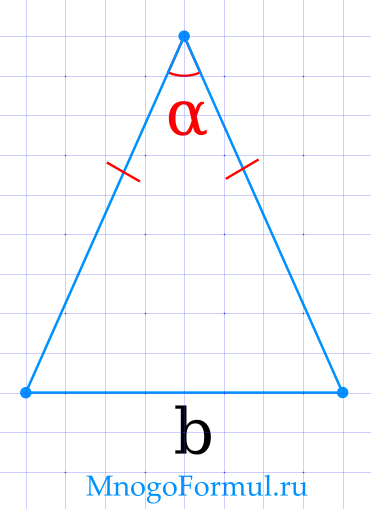- the height drawn to the base.

The area of ​​an isosceles triangle through the sides and the angle between them

- the height drawn to the base. You can always check the correctness of calculating the area of ​​a triangle using our calculator. bwhere α{S = \ dfrac {1} {2} \ cdot b \ cdot h}

## Formula for the area of ​​an isosceles triangle in terms of the sides and the angle between them:- the angle between the sides.

Area of ​​an isosceles triangle through the base and the angle between the sides

- the angle between the sides. You can always check the correctness of calculating the area of ​​a triangle using our calculator. RFormula for finding the area of ​​a triangle in terms of base and height:

## {S = \ dfrac {b ^ 2} {4 \ cdot tg \ dfrac {\ alpha} {2}}}Formula for the area of ​​an isosceles triangle in terms of the base and the angle between the sides:

Equilateral triangle area

Formula for the area of ​​an isosceles triangle in terms of the base and the angle between the sides: You can always check the correctness of calculating the area of ​​a triangle using our calculator. r{S = \ dfrac {a \ cdot b \ cdot c} {4 \ cdot R}}

## The area of ​​an equilateral triangle through the radius of the circumscribed circle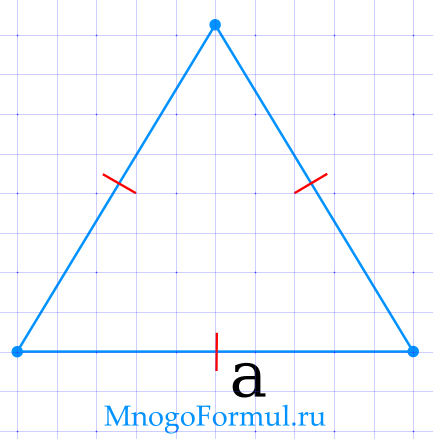{S = \ dfrac {3 \ sqrt {3} \ cdot R ^ 2} {4}}

Formula for the area of ​​an equilateral triangle in terms of the radius of the circumscribed circle:

{S = \ dfrac {3 \ sqrt {3} \ cdot R ^ 2} {4}} You can always check the correctness of calculating the area of ​​a triangle using our calculator. aThe area of ​​an equilateral triangle through the radius of the inscribed circle

## {S = 3 \ sqrt {3} \ cdot r ^ 2}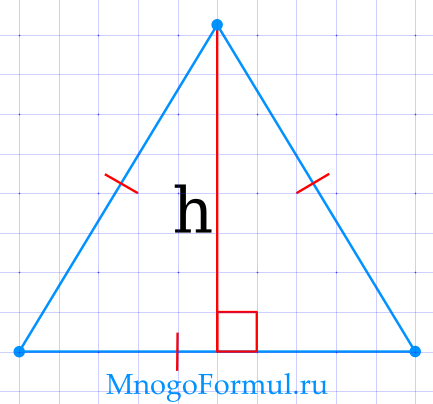Formula for the area of ​​an equilateral triangle in terms of the inscribed circle radius:

Equilateral triangle area across side

Formula for the area of ​​an equilateral triangle in terms of the inscribed circle radius: You can always check the correctness of calculating the area of ​​a triangle using our calculator. h- sides of a triangle,

{S = \ dfrac {\ sqrt {3} \ cdot a ^ 2} {4}} Formula for the area of ​​an equilateral triangle in terms of the side:

Is the side of the triangle.

Equilateral triangle area in terms of height

## {S = \ dfrac {h ^ 2} {\ sqrt {3}}}

Formula for the area of ​​an equilateral triangle in terms of height:

Page views: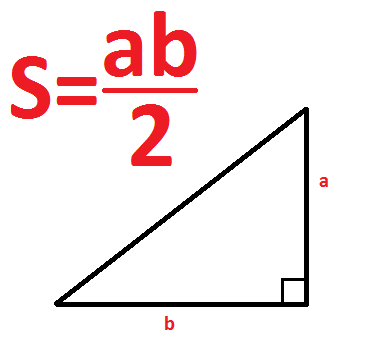## 327423

Depends on which triangle.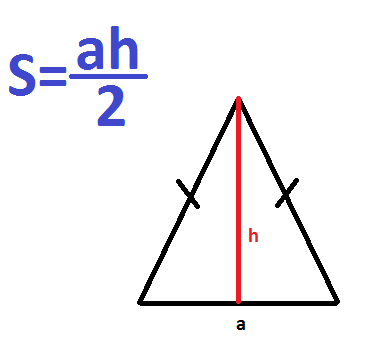## To find the area of ​​a triangle, you must first determine the type of triangle: right-angled, isosceles, equilateral. If you have it differently, start from other data: the height, inscribed or circumscribed circle, the lengths of the sides. I present all the formulas below.

If the triangle is rectangular

1. That is, one of its angles is 90 degrees.
2. It is necessary to multiply the legs and divide by two. The legs are the two smaller sides compared to the hypotenuse. The hypotenuse is the longest side and is always opposite a 90 degree angle.
3. If he is isosceles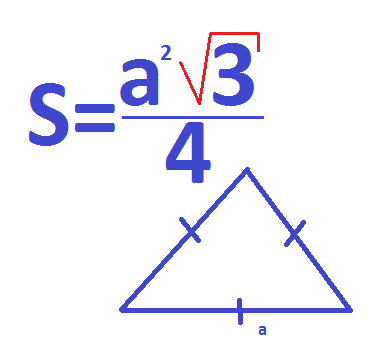## That is, it has equal sides. In this case, you need to draw the height to the base (the side that is not equal to the "hips"), multiply the height with the base and divide the result by two.

If it's equilateral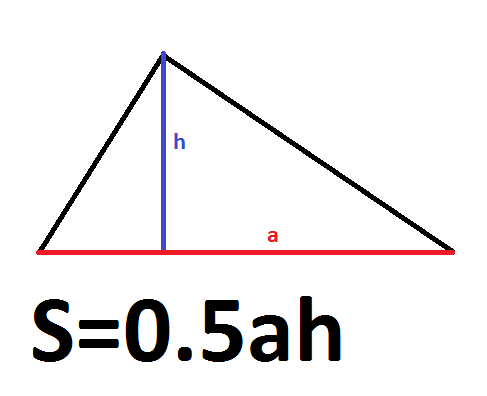That is, all three sides are equal. Your actions are as follows:

## Find the square of a side - multiply that side by that side. If your side is 4, multiply 4 by 4, that's 16.

Multiply this by the root of 3. This is approximately 1.732050807568877293527.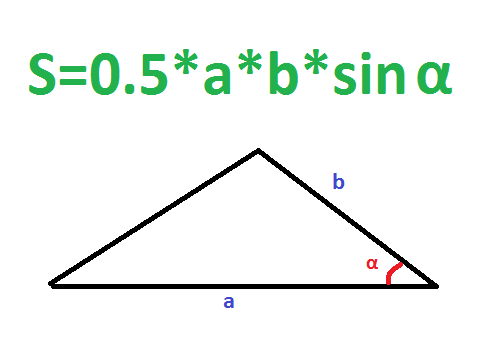## Divide everything by 4.

If side and height are known

1. The area of ​​any triangle is equal to half the product of a side by the height that is drawn to this side. It is to this one, and not to some other.
2. To draw the height to a side, you need to find the vertex (angle) that is opposite to this side, and then lower a straight line from it to the side at an angle of 90 degrees. In the picture, the height is indicated in blue and the letter h, and the line to which it falls, in red and the letter a.
3. If two sides and the degree of the angle between them are known
4. If you know what the two sides are and the angle between them, then you need to find the sine of this angle, multiply it by the first side, multiply by the second and multiply by ½:
5. If the lengths of the three sides are known
6. Do this:
7. Find the perimeter. To do this, fold all three sides.Find a semi-perimeter - divide the perimeter in two. Remember the meaning.

## Subtract the length of the first side from the semi-perimeter. Remember.

Subtract the length of the second side from the semi-perimeter. Remember too.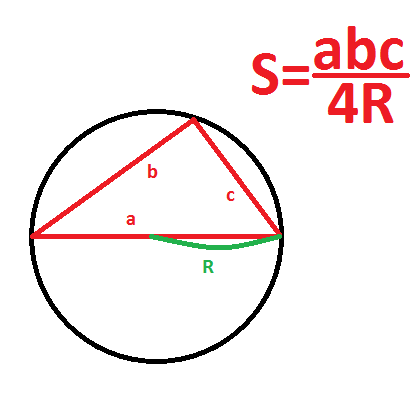## Subtract the length of the third side from the semi-perimeter. And remember it.

Multiply the half-perimeter by each of these numbers (the difference with the first, second, and third sides).

Find the square root.This formula is also called Heron's formula. Take note if the teacher asks.

## If three sides and radius of the circumscribed circle are known

You can describe a circle around any triangle. To find the area of ​​the "inscribed" triangle - the one that "fit" into the circle, you need to multiply its three sides and divide them by four radii. See the picture.

а

If the three sides and the radius of the inscribed circle are known

If you managed to inscribe a circle in the triangle, then it necessarily touches each of its sides. Therefore, the distance from the center of the circle to each side of the triangle is its radius.

To find the area, first count the semi-perimeter - fold all sides and divide by two. And then multiply it by the radius.

• These were all ways to find the area of ​​a triangle. Thank you for reading the article to the end. Like if it's not difficult. 2Basic concepts
• A triangle is a geometric shape that is made up of three line segments. They were connected by three points that did not lie on one straight line. The segments are called sides, and points are called vertices. 2Basic concepts
• Area is a numerical characteristic that gives us information about the size of a plane bounded by a closed geometric figure. 2Basic concepts
• If the parameters are passed in different units of length, we will not be able to know how much of the triangle will be. Therefore, for the correct solution, it is necessary to convert all data to one unit of measurement. 2Basic concepts
• Popular units 2Basic concepts
• square millimeter (mm

## );

square centimeter (cm

## square decimeter (dm

### square meter (m

square kilometer (km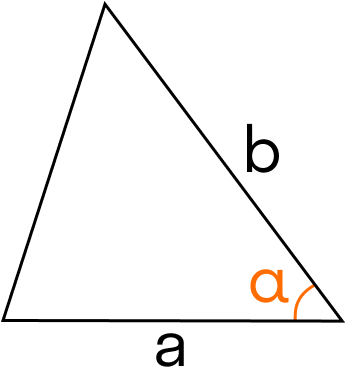### hectare (ha).

The formula for the area of ​​a triangle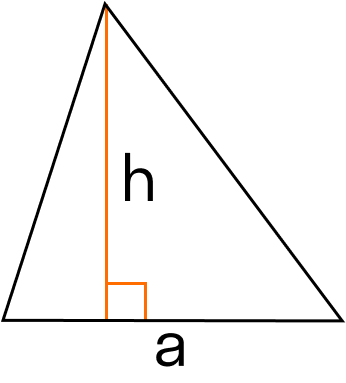### Various formulas are used to solve problems, depending on the known initial data. Next, we will consider ways to solve for all types of triangles, including special cases for equilateral, isosceles and rectangular shapes.

General formula### 1. Area of ​​a triangle through two sides and the angle between them.

S = 0.5 * a * b⋅sin (α), where a, b are sides, α is the angle between them.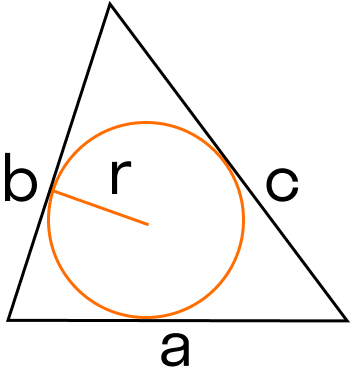2. The area of ​​the triangle through the base and height.

S = 0.5 * a * h, where a is the base, h is the height.

### 3. Area of ​​a triangle through the circumcircle and sides.

S = (a * b * c): (4 * R), where a, b, c are sides, R is the radius of the circumscribed circle. 24. The area of ​​the triangle through the inscribed circle and sides.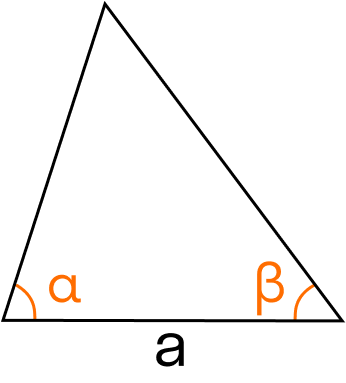### S = r * (a + b + c): 2, where a, b, c are sides, r is the radius of the inscribed circle.

Considering that (a + b + c): 2 is a way to find a semi-perimeter. Then the formula can be written as follows:

S = r * p, where p is a semiperimeter.## 5. Area of ​​a triangle along a side and two adjacent corners.

### S = a

: 2 * (sin (α) ⋅sin (β)): sin (180 - (α + β)), where a is the side, α and β are the adjacent angles, γ is the opposite angle.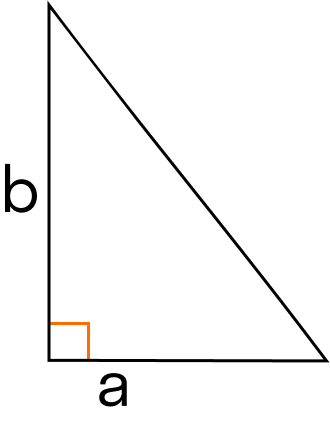### 6. Heron's formula for calculating the area of ​​a triangle.

First, you need to calculate the difference between the semi-perimeter and each side. Then find the product of the numbers obtained, multiply the result by a semi-perimeter and find the root of the resulting number. 2S = √ p * (p - a) * (p - b) * (p - c), where a, b, c are sides, p is a semiperimeter, which can be found by the formula: p = (a + b + c): 2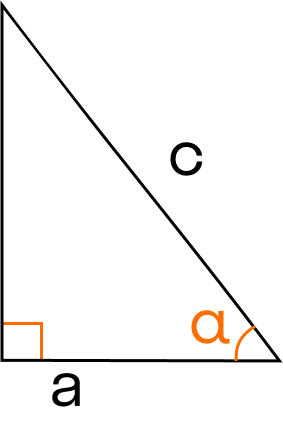For a right triangle

### Area of ​​a triangle with an angle of 90 ° on two sides.

S = 0.5 * a * b, where a, b are sides. 2Hypotenuse and acute angle area of ​​a triangle.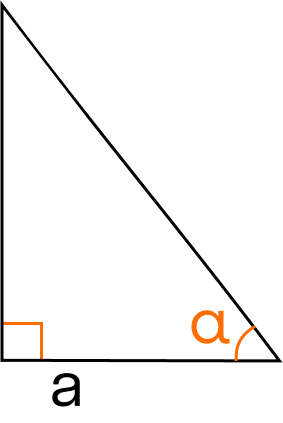S = 0.25 * s

### * sin (2α), where c is the hypotenuse, α is any of the adjacent acute angles.

The hypotenuse is usually called the side that lies opposite the right angle.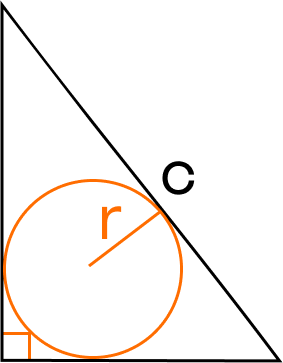### The area of ​​a right-angled triangle along the leg and the adjacent corner.

S = 0.5 * a 1* tg (α), where a - leg, α - included angle. 2A leg is usually called one of the two sides forming a right angle. 1Area of ​​a triangle through the hypotenuse and along the radius of the inscribed circle. 2{S = r \ cdot (r + c)}### S = r * (r + c), where c is the hypotenuse, r is the radius of the inscribed circle.

Area of ​​a triangle inscribed in a circle.## S = c

### * c

where c 2, c 2Area of ​​a right triangle according to Heron's formula.### S = (p - a) * (p - b), where a, b - legs, p - semi-perimeter, which is calculated by the formula p = (a + b + c): 2.

For an isosceles triangle### Search for the area through the base and side.

S = b: 4 * √ 4 * a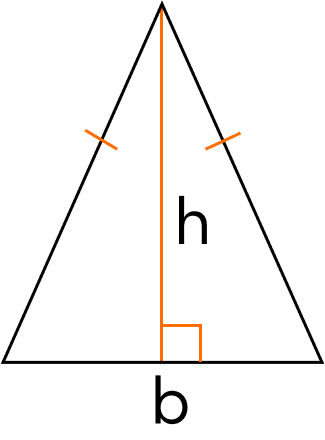### - b

S = 0.5 * a * b, where a, b are sides. 2, where a - side, b - base.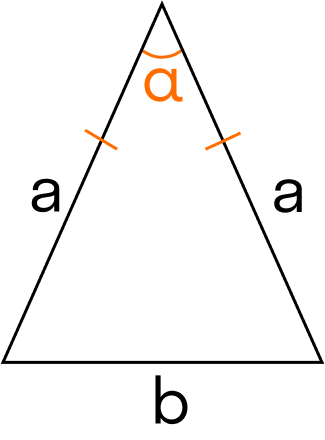### Calculating area using base and angle.

S = 0.5 * a * b * sin (α), where a is the side, b is the base, α is the angle between the base and the side. 2Calculating area through base and height.### S = 0.5 * b * h, where b is the base, h is the height drawn to the base.

Search for the area through the sides and the angle between them. 2* sin (α), where a is the lateral side, α is the angle between the lateral sides.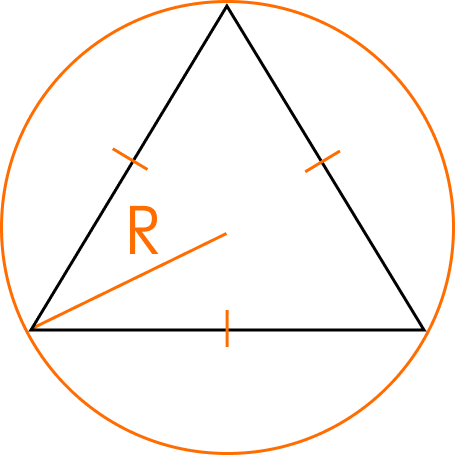### Area of ​​an isosceles triangle through the base and the angle between the sides.

S = b 2: (4 * tgα / 2), where b is the base, α is the angle between the sides.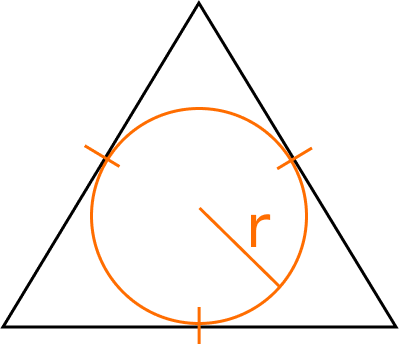### The area of ​​an equilateral triangle through the radius of the circumscribed circle.

S = (3 * √ 3 * R 2): 4, where R is the radius of the circumscribed circle.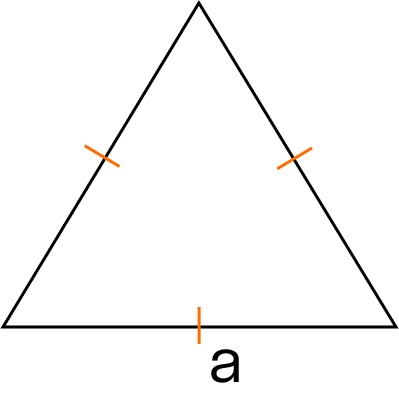### The area of ​​an equilateral triangle through the radius of the inscribed circle.

S = 3 * √ 3 * r 2, where r is the radius of the inscribed circle.The area of ​​an equilateral triangle across the side.S = (√ 3 * a

): 4, where a is a side.